# Electronics and Communication Engineering - Exam Questions Papers

26.

Consider the signal flow graph shown in figure below. The gain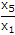is :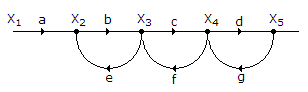A.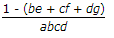B.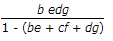C.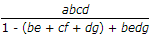D.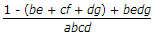Answer: Option C

Explanation:

P1 = abcd

L1 = be, L2 = cf, L3 = dg

Δ1 = 1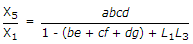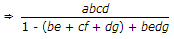.

27.

During transmission over a communicate channel, bit errors occur independently with probability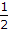. If a block of 3 bits are transmitted, the probability of at most one bit error is equal to

 A.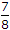B.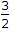C.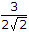D. none

Answer: Option D

Explanation:

Probability of no-error + probability one error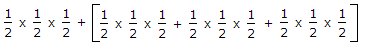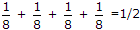.

28.

If x1[n]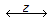x1(z) with ROC = R1 and x2[n]x2(z) with ROC = R2 then ROC for x1[n] + x2[n] will be __________ (where Ri → Region of convergence and x1[n] and x2[n] are causal).

 A. R1 ∩ R2 B. R1 ∪ R2 C. (R1 ∪ R2) ∩ (R1) D. (R1 ∩ R2) ∪ (R1)

Answer: Option A

Explanation:

R1 ∩ R2, Suppose R1 for x1(n) be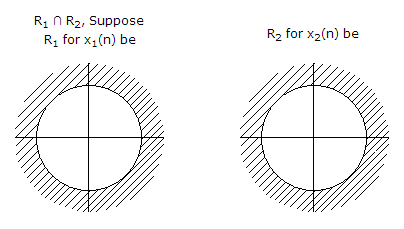Then ROC for x1(n) + x2(n)

R1 ∩ R2 (R1 < R2)

Then ROC for x1(n) + x2(n)

R1 ∩ R2(R1 < R2)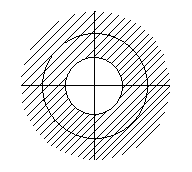29.

Consider a unity feedback control system with open-loop transfer function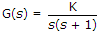The steady state error of the system due to a unity step input is :

 A. zero B. K C. 1/K D. infinite

Answer: Option A

Explanation: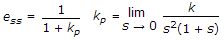.

30.

The analog signal given below is sampled at 1200 samples per second. m(t) = 2 sin 120 pt + 3 sin 240 pt + 4 sin 360 pt + 5 sin 480 pt + 6 sin 600 pt + 7 sin 720 pt. The folding (maximum) frequency is __________ .

 A. 300 Hz B. 360 Hz C. 600 Hz D. 480 Hz

Answer: Option C

Explanation:

Folding frequency (max.) =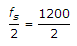= 600 Hz.

#### Current Affairs 2021

Interview Questions and Answers